# Optimal solution for circuit design

2020-11-30

Basic lecture

Technical information

## Basic Knowledge of Thermal Management (1) -Basic Knowledge of Heat-

Download this article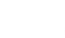### Introduction

Thermal management is an important process for ensuring the characteristics, life, and safety of equipment and systems. Basic knowledge for carrying out necessary thermal management will be explained in three parts, "Basic Knowledge of Heat," "Background to the Need of Thermal Management," and "Thermal Design and Management." First, this issue will explain heat-related definitions, terms, etc.

### Basic Knowledge of Heat

In order to carry out thermal management, it is necessary to know the definition of heat, i.e., the basics of heat, such as its characteristics, definitions, and units.

1. Temperature and Heat

Temperature is a measure that indicates the hotness and coldness of objects. The Celsius temperature scale uses the unit symbol °C, whereas the Fahrenheit temperature scale uses °F. Using these scales, temperature can indicate, for example, that bath water at a temperature of 42°C is hotter than that at 38°C. Although dictionaries and other references also give thermodynamic definitions, please understand temperature as a measure of the degree of hot and cold here.

Heat is energy that transfers from a high temperature section to a low temperature section, changing the temperature of an object by entering or exiting into/from it. For example, pouring hot water into a cup makes the cup hot. This is because the heat of the hot water (approx. 100° C) = energy transfers to the cup (e.g. room temperature).

2. Heat Quantity, Joule

Heat quantity is a measure of the energy flow between objects, i.e. the quantification for amount of heat. It uses the symbol Q (Q of heat Quantity) and the unit J (joule).

Joule is a unit that indicates the amount of energy required for work to move an object by applying a force to it. Joule is also used as a unit of work, heat quantity, and amount of electric power, in addition to the amount of energy. For example, 1 J can be converted to 1 N・m (newton meter) for work, which has the same meaning.

3. Amount of Heat Generation

The amount of heat generation refers to the amount of generation or transfer of heat per unit time. While the unit of heat quantity is joule (J), watt (W) is mainly used for the amount of heat generation. Watt is defined by joule and time (sec).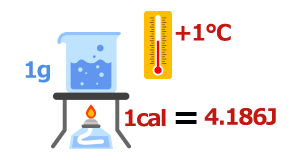W＝J/s
W
Amount of heat generation
J
Amount of energy
s
Time (sec)

By the way, the basic definition of "calorie," a unit of heat quantity, is the "amount of heat required to raise the temperature of 1 g of water by 1°C under the standard atmospheric pressure." 1 calorie is 4.184 J.

4. Specific Heat and Heat Capacity

Specific heat refers to the amount of heat required to raise the temperature of 1 g of a substance by 1°C.
It uses the symbol "c" and the unit J/K・g. (K: absolute temperature (+1°C = +1 K)).
Specific heat differs by substance.

Heat capacity refers to the amount of heat required to raise the temperature of a specific amount of substance by 1°C. It uses the symbol C, and its value is obtained by multiplying the specific heat of the substance by the number of grams.

5. Properties of Heat: Heat Transfer (Heat Transmission), Thermal Equilibrium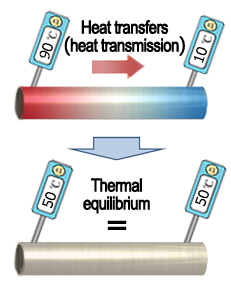Two important properties of heat are described below.

• Heat always transfers from a substance on a higher temperature side to a substance on a lower temperature side.
Heat transfer (heat transmission)
• When the temperatures of both substances become equal, no more heat is transferred.
Thermal equilibrium

Perhaps everyone can visualize these concepts from their everyday experiences. Heat transferring from a higher to a lower temperature side is referred to as heat transfer or heat transmission. When the temperatures of both sides become the same, the heat transfer stops, which is referred to as a thermal equilibrium state.

6. Types of Heat Transfers

There are three types of heat transfers (heat transmission): Heat conduction and convection in which a substance carries heat, and heat radiation in which heat is transferred by electromagnetic waves. The drawing of a fire clearly shows each type of transfer. The heat generated by the fire is conveyed by a metal bar as a medium (heat conduction), and reaches the hands through the rising warm air (convection), and also reaches the hands through radiation (heat radiation).

#### Heat Conduction

Heat conduction (conduction) refers to the phenomenon in which heat is carried (conveyed) by a substance. Heat is carried by the propagation of the lattice vibration of atoms/molecules and the movement of free electrons.

High temperature

Low temperature

gases <  liquid <  solids

Numerical values that indicate this ease of conduction are referred to as thermal conductivity.
Thermal conductivity indicates the amount of heat that transfers through a unit cross-sectional area 1m2 in a unit time 1 sec when there is a temperature difference of 1°C per meter of length, and its unit is (W/m・K). Higher numerical values of thermal conductivity mean that heat is conducted more easily. Thermal conductivity differs by substance. Heat is conducted more easily in the order of gases <  liquid <  solids, as described earlier, which means that thermal conductivity is higher in the order of gases <  liquid <  solids. Thermal conductivity naturally differs by type of solid.

The diagram below shows the thermal conductivities of various materials.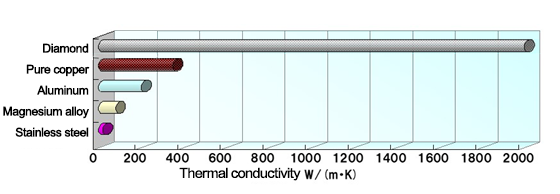#### Convection

Convection is a phenomenon in which heat is carried by the movement of a fluid (gas or liquid) due to temperature differences. When the temperature rises, gases and liquids expand, become less dense and lighter, and rise as a result. Conversely, when temperature falls, they become heavier and drop.

High temperature

Low temperature

Heat radiation is a phenomenon in which heat is carried by radiation (electromagnetic waves). Heat can even be conveyed in a vacuum because this is not a heat transfer via a substance but via radiation. The heat of the sun reaches the earth by way of this heat radiation mechanism.#### Roles of Heat Conduction, Convection, and Heat Radiation in the Cooling of Equipment

This diagram shows how conduction, convection, and heat radiation are related to the cooling of equipment and components.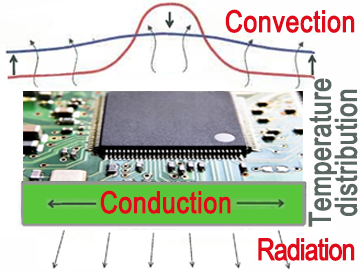Heat conduction: Contributes to temperature equalization
Convection: Contributes to the reduction of average temperature (approx. 80% of heat dissipation)
Heat radiation: Auxiliary role of convection (approx. 20% of heat dissipation)

In this example, the heat generated by the electronic component is transferred to the mounting board by heat conduction and dispersed. The heat of the component and mounting board cause convection, lowering the temperature by averaging the ambient temperature. In this case, the heat radiation of the mounting board plays an auxiliary role of convection.

### Summary

The basics for carrying out thermal management have been explained with a focus on the basic characteristics of heat and related terms. characteristics, etc.
Since heat is a relatively familiar presence, everyday experiences, etc. may help you visualize its

The meanings of terms were briefly described with a focus on important points. Please also see dictionaries, etc. if details are necessary. The next issue will explain why thermal management is important, including its background, etc.

↑Page top